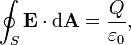Home » Posts tagged 'gauss’s law'

# Tag Archives: gauss’s law

## Explain a method to create Uniform Electric Field.

The simplest method of creating a uniform electric field in a region is to keep two  equally charged thin plain metal sheets of opposite charge parallel to each other. As you can see from the derivation for electric field due to a plain sheet of charge, the electric field due to a plain sheet of charge is independent of distance (provided the distance is not large compared to the dimensions of the plain sheet of charge).

So, if you keep two plain metal sheets of same charge densities but opposite kind of charge, we get a fairly good uniform electric field in between the plates.

At regions close to a uniformly charged thin sheet also we get a uniform electric field, but as distance increases it may tend to become non uniform.## Gauss`s law

“A circular ring of radius r made of a non conducting material is placed with its axis is parallel to a uniform electric field if the ring rotated by 180 degrees Does the flux changes? A Q charge is uniformly distributed on a thin spherical shell If a point charge is brought near it what is the field at the center?Is u r answer depends on whether it is conducting or non conducting?”

## Gauss Theorem – Electrostatics

“WHAT IS THE  MAGNITUDE OF ELECTRIC  FIELD IN THE  GAUSSIAN SURFACE OF  A CUBE,AT ITS FACE ,AT ITS CENTRE, AT ITS CORNERS OR AT ANY OTHER POINT INSIDE THE CUBE. ALSO TELL ME THE THE WAY TO KNOW IT.”

Ans:

According to Gauss Theorem, the total electric flux through a closed surface,The Gaussian surface is an imaginary surface. So, for calculating the electric field at a point using Gauss theorem, we have to imagine a Gaussian surface symmetric with the given charge distribution.

I have assumed that there is a point charge Q at the centre of the cube.

At its face (at a point on the face on the line radially outwards from the point charge at the centre of the cube and perpendicular to the face)

The distance is equal to half the length of side of the cube (Say L).

On the corner,

Calculate the distance from the centre of the cube to its corner and replace (L/2) in the above equation with that distance.

## A Question from Electric Flux and Gauss Theorem

How can you prove that dS in φ =E.dS is dS Cos θ if the area is tilted at an angle ? I need the mathematical Steps.

Ans;

Dear Zeenath,
Electric Flux is defined as the total no of field lines passing normal to the surface. So while calculating, we need to consider the area of the surface normal to the field lines only. That is why we take the dot product of E and ds, where ds is the area vector (not just the area: Area vector is a vector whose magnitude is equal to the area and dirtected normal to the surface.)
Then by definition of dot product,
dφ =E.dS = EdS cos θ
which gives the component of E and the component of area vector in the direction of E (When area vector is in the direction of E, the actual component of area is perpendicular to E)
Hope the matter is clear.
Mathew Abraham

### Visitors So Far @ AskPhysics

• 2,168,058 hits

### Subscribe to Blog via EmailAsk Physics